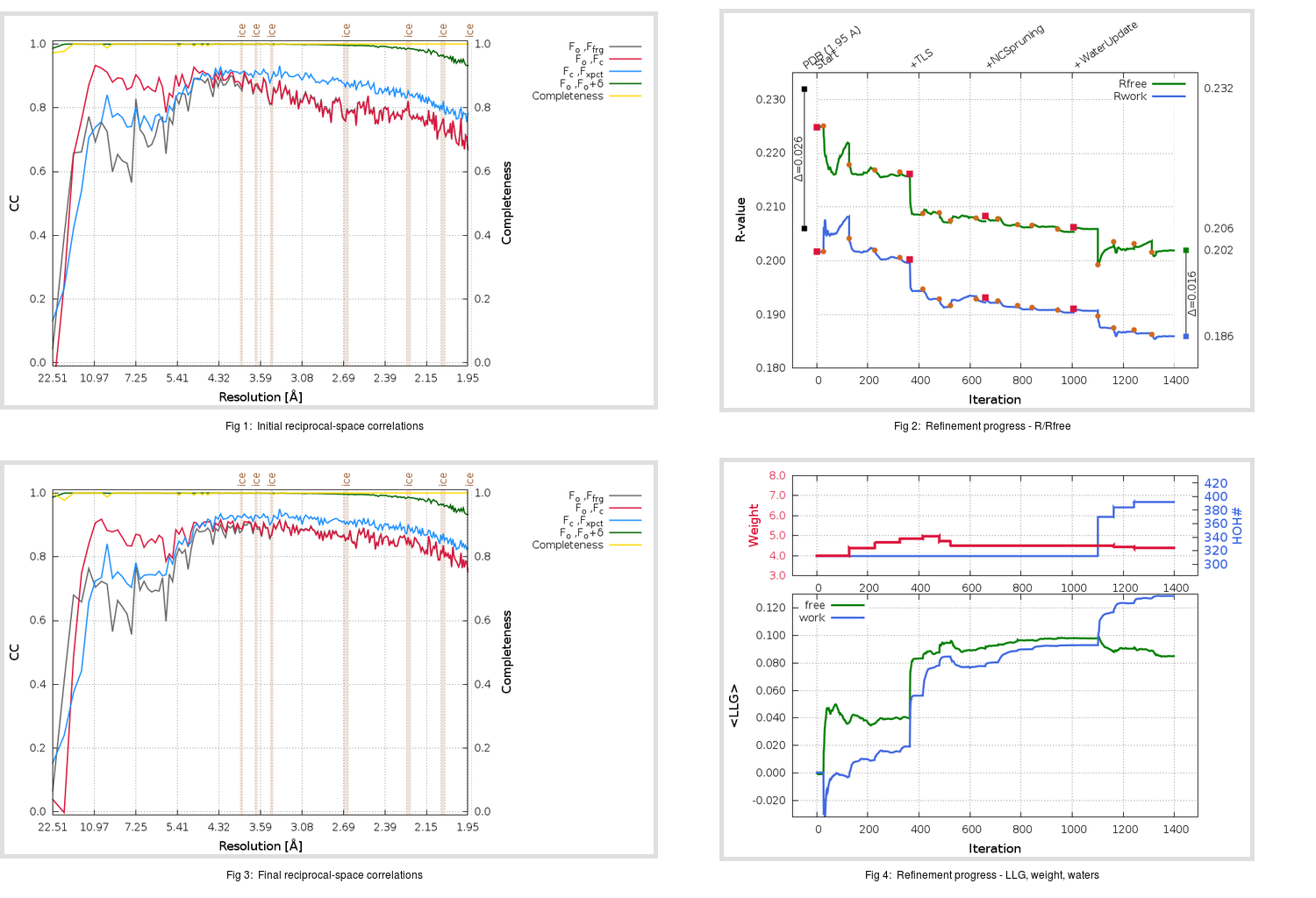Content:

```    Diffraction limits & principal axes of ellipsoid fitted to diffraction cut-off surface:
1.906         1.0000   0.0000   0.0000       0.959 a* - 0.285 c*
1.920         0.0000   1.0000   0.0000       b*
1.917         0.0000   0.0000   1.0000       c*
```

## Deposited

` `
 Date deposited Date data collection Resolution R, Rfree 20111231 20091012 1.95 0.2040 0.2320

Molprobity (CCP4 7.0 version) summary:

```Ramachandran outliers =   0.66 %
favored =  95.70 %
Rotamer outliers      =   2.27 %
C-beta deviations     =     1
Clashscore            =   8.60
RMS(bonds)            =   0.0073
RMS(angles)           =   1.22
MolProbity score      =   2.03
Resolution            =   1.95
R-work                =   0.2040
R-free                =   0.2320
```

```Number of waters      =   312

<B> (all atoms) =   30.86 ( sd =   11.94 ) for       5122 non-hydrogen atoms
<B>   (protein) =   30.40 ( sd =   12.02 ) for       4760 non-hydrogen atoms
<B>     (water) =   36.01 ( sd =    8.34 ) for        312 non-hydrogen atoms
<B>    (others) =   42.66 ( sd =   10.04 ) for         50 non-hydrogen atoms

B min/max       (all non-hydrogen atoms) =   11.76 /   95.44
B min/max   (protein non-hydrogen atoms) =   11.76 /   95.44
B min/max     (water non-hydrogen atoms) =   16.68 /   57.57
B min/max     (other non-hydrogen atoms) =   23.54 /   63.28
```

## BUSTER (re-)refinement

` `

Molprobity (CCP4 7.0 version) summary:

```Ramachandran outliers =   0.00 %
favored =  98.18 %
Rotamer outliers      =   1.89 %
C-beta deviations     =     0
Clashscore            =   2.62
RMS(bonds)            =   0.0113
RMS(angles)           =   1.54
MolProbity score      =   1.26
Resolution            =   1.95
R-work                =   0.1860
R-free                =   0.2019
```

```Number of waters      =   392

<B> (all atoms) =   32.42 ( sd =   11.93 ) for       5202 non-hydrogen atoms
<B>   (protein) =   31.49 ( sd =   11.49 ) for       4760 non-hydrogen atoms
<B>     (water) =   41.97 ( sd =   12.28 ) for        392 non-hydrogen atoms
<B>    (others) =   46.06 ( sd =    7.36 ) for         50 non-hydrogen atoms

B min/max       (all non-hydrogen atoms) =   14.61 /  145.72
B min/max   (protein non-hydrogen atoms) =   14.61 /   92.77
B min/max     (water non-hydrogen atoms) =   16.30 /  145.72
B min/max     (other non-hydrogen atoms) =   33.26 /   56.63
```

Refinement progression:Results:

` `
 File Remark 3VB5_aB_refine.01_04_refine.pdb.gz exact refinement commands are in header 3VB5_aB_refine.01_04_refine.mtz.gz including original deposited data and several re-refinement map coefficients 3VB5_aB_refine.01_04_BUSTER_model.cif.gz including any non-standard compound restraints 3VB5_aB_refine.01_04_BUSTER_refln.cif.gz